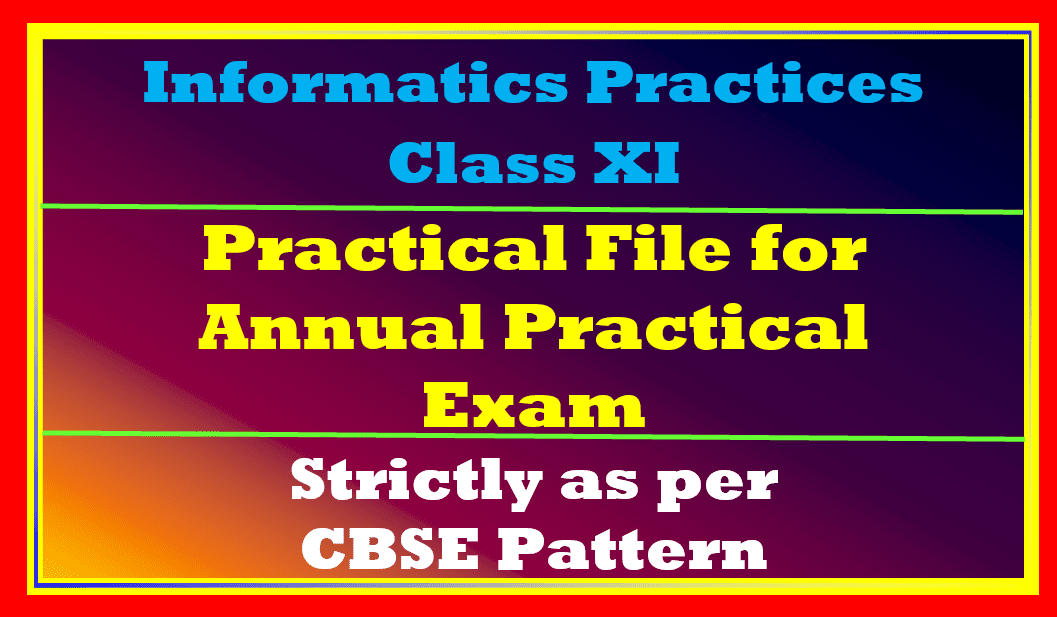In this article, I will discuss Practical File Informatics Practices Class 11. This is practical file is strictly prepared as per syllabus of Informatics Practices for session 2022-23. So here we begin!

Topics Covered

## Practical File Informatics Practices Class 11

The syllal has a python and database management system in the practical. To create Practical File Informatics Practices Class 11 you need to follow the practical assessment structure suggested by CBSE.

### Practical Assessment Structure

It is as follows:

As per the above table, you need to write 14 python programs and 14 MySQL queries for Practical File Informatics Practices Class 11. In the next section, we will see the suggested programs and queries given in your syllabus.

## Suggested Practical List Practical File Informatics Practices Class 11

The suggested programs given in the syllabus for Informatics Practices Class 11 are as follows:

### Python programs for class 11 Practicals

In CBSE syllabus the suggested MySQL queries will be given, they are as follows:

### Suggested MySQL Queries for class 11 IP practicals

1. To create a student table with the student id, class, section, gender, name, dob, and marks as
attributes where the student id is the primary key.
2. To insert the details of at least 10 students in the above table.
3. To delete the details of a particular student in the above table.
4. To increase marks by 5% for those students who have Rno more than 20.
5. To display the entire content of the table.
6. To display Rno, Name and Marks of those students who are scoring marks more than 50.
7. To find the average of marks from the student table.
8. To find the number of students, who are from section ‘A’.
9. To add a new column email in the above table with appropriate data type.
10. To add the email ids of each student in the previously created email column.
11. To display the information of all the students, whose name starts with ‘AN’ (Examples: ANAND,
12. To display Rno, Name, DOB of those students who are born between ‘2005- 01-01’ and
‘2005-12-31’.
13. To display Rno, Name, DOB, Marks, Email of those male students in ascending order of their
names.
14. To display Rno, Gender, Name, DOB, Marks, Email in descending order of their marks.
15. To display the unique section available in the table.

So let us begin now Practical File Informatics Practices Class 11. I will cover all the queries given above and few more additional queries as per given in the practical structure. So let us start now!

## Python Practical Programs for Practical File Informatics Practices Class 11

So let us begiin with MySQL quries now. Here we go!

## MySQL queries for Practical File Informatics Practices Class 11

 Create Database

Create a database as given in the suggested practical list. There is no name provided in the practical list. So I have created a database named Class11. The create database command is used to do so.

``create database class11;``

 Open database

``use class11;``

 To create a student table with the student id, class, section, gender, name, dob, and marks as
attributes where the student id is the primary key.

``````create table student
(studentid int(4) primary key,
class char(2),
section char(1),
gender char(1),
name varchar(20),
dob date,
marks decimal(5,2));``````

 View the structure of the table

``````desc student; OR
describe student``````

Note: Use any of one command for your practical file.

 To insert the details of at least 10 students in the above table.

``````insert into student values
(1101,'XI','A','M','Aksh','2005/12/23',88.21),
(1102,'XI','B','F','Moksha','2005/03/24',77.90),
(1103,'XI','A','F','Archi','2006/04/21',76.20),
(1104,'XI','B','M','Bhavin','2005/09/15',68.23),
(1105,'XI','C','M','Kevin','2005/08/23',66.33),
(1107,'XI','D','M','Krish','2005/01/23',84.33),
(1108,'XI','D','M','Ayush','2005/04/23',55.33),
(1109,'XI','C','F','Shruti','2005/06/01',74.33),
(1110,'XI','D','F','Shivi','2005/10/19',72.30);``````

 Display the details of the student table.

``select * from student;``

 To delete the details of a particular student in the above table.

Delete record of students who secured less than 65 marks.

``delete from student where marks <65;``

 To increase marks by 5% for those students who have studentid more than 1105.

``update stduent set marks=makrs+(marks*0.05) where studentid>1105;``

 To display the content of the table of female students.

``select * from student where gender = 'f';``

 To display studentid, Name and Marks of those students who are scoring marks more than 50.

``select studentid,name,marks from student where marks>50;``

 To find the average of marks from the student table.

``select avg(marks) from student;``

 To find the number of students, who are from section ‘A’.

``select count(*) from student where section = 'A';``

 To add a new column email in the above table with the appropriate data type.

``alter table student add column email varchar(20);``

 To add the email ids of each student in the previously created email column.

``update student set email='a@a.com';``

 To display the information of all the students, whose name contains ‘sh’

``select * from student where name like 'sh%';``

 To display the information of all the students, whose name starts with ‘sh’

``select * from stduent where name like 'sh%';``

 To display studentid, Name, DOB of those students who are born between ‘2005- 01-01’ and
‘2005-12-31’.

``select studentif, name, dob from student where dob beetween '2005-01-01' and '2005-12-31';``

 To display studentid, Name, DOB, Marks, Email of those male students in ascending order of their
names.

``select studentid, name, dob from student order by name;``

 To display stduentid, Gender, Name, DOB, Marks, Email in descending order of their marks.

``select studentid, gender, name, dob, marks, email from student order by marks desc;``

 To display the unique section available in the table.

``select distnict section from student;``

Watch this video for more clarity:

1.Harkesh says: